Courses

# Analog Electronics - 1

## 10 Questions MCQ Test RRB JE for Electronics & Communication Engineering | Analog Electronics - 1

Description
This mock test of Analog Electronics - 1 for Electronics and Communication Engineering (ECE) helps you for every Electronics and Communication Engineering (ECE) entrance exam. This contains 10 Multiple Choice Questions for Electronics and Communication Engineering (ECE) Analog Electronics - 1 (mcq) to study with solutions a complete question bank. The solved questions answers in this Analog Electronics - 1 quiz give you a good mix of easy questions and tough questions. Electronics and Communication Engineering (ECE) students definitely take this Analog Electronics - 1 exercise for a better result in the exam. You can find other Analog Electronics - 1 extra questions, long questions & short questions for Electronics and Communication Engineering (ECE) on EduRev as well by searching above.
QUESTION: 1

### A half-wave rectifier is used to charge a 12V battery through a resistance ‘R’. The input transformer is fed with 34 AC with turns ration 2 : 1. The conduction period of the diode is

Solution: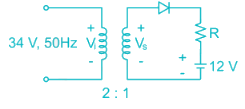Voltage at secondary winding of the coil is Vs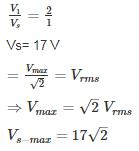Voltage across diode

Vp → Vs max

Vn → 12 V

For conduction:

Vp > Vn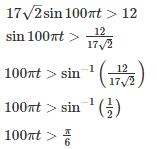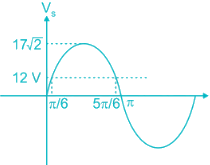Therefore the diode is conducting for the period.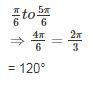*Answer can only contain numeric values
QUESTION: 2

### A full-wave rectifier circuit employs inductor filter at its output. If the source frequency is 50 Hz and the inductor value is 10 mH. Find the ripple factor for 100 Ω loads.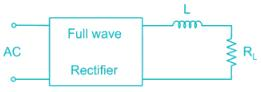Solution:

For full wave rectifier using inductor filter at output is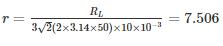*Answer can only contain numeric values
QUESTION: 3

### The voltage source VAB = 4 sin ωt is applied to the terminal A and B of the circuit shown In Fig. the diode is assumed to be ideal. The impedance by the circuit across the terminals A and B is ________ kΩ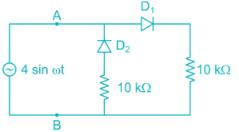Solution:

When,

D1 → ON; D2 → OFF: RAB = 10 kΩ

D2 → ON; D1 → OFF: RAB = 10 kΩ

∴ The impedance by the circuit across the terminals A and B  of 10 kΩ

QUESTION: 4

For the damping network shown below, resulting output for the applied Input will be…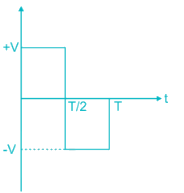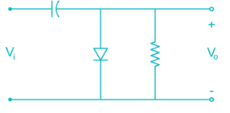Solution:

Clamping network “Clamp” a signal to different DC level.

During the Internal O → T/1 → Diode in the ON state during this internal output voltage is directly across the short circuit and Vo = 0 volts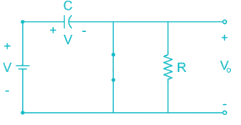When input switch to -V  state → diode OFF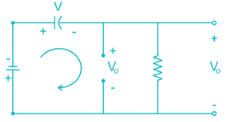Applying Kirchhoff’s voltage law around loop we get

-V -V -Vo = 0

Vo = -2V

So output wave form will be,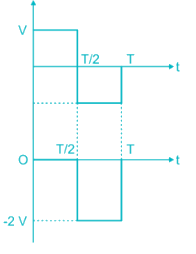*Answer can only contain numeric values
QUESTION: 5

Assuming the diode to be ideal, the current I in the circuit shown is ________ mA.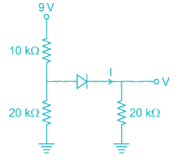Solution:

The above circuit can be redrawn as: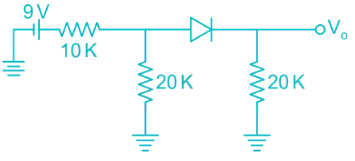Assume diode is off:

Voltage across 20 k resistor connected to left of diode is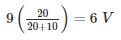This will turn on the diode

the equivalent circuit is now: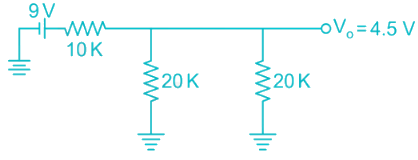Now the two 20k resistors are in parallel whose equivalent is 10k

this 10 k is in series with other 10k resistor

9V divides equally among them

V0 = 4.5V

Cuurent I = 4.5/20 mA

= 0.225 mA

QUESTION: 6

The correct option matching List - I to List - II is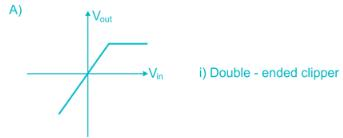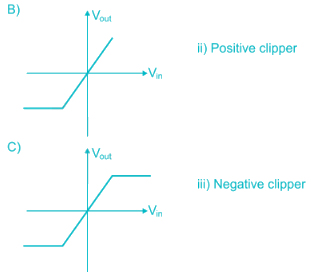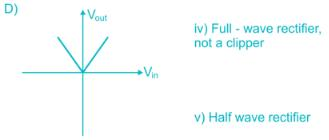Solution:

A) In graph ‘A’ the output follows input, but above certain positive input, output stays constant. The circuit is called positive clipper.

B) Similarly the graph ‘B’ is of negative clipper.

C) In this path positive and negative side are clipped, it is a double-ended clipper.

D) In this input output follows relation Vout = |Vin|. This is a full-wave rectifier circuit.

*Answer can only contain numeric values
QUESTION: 7

The figure shows a half wave rectifier with a load resistor of 50 Ohms. The input supply voltage is 230V(runs) at 50 Hz. A 10000 μF filter capacitor is added across the load resistor to reduce ripple. The percentage of ripple voltage at the output is ________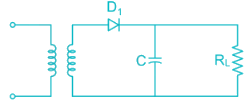Solution:

Due to filter capacitor the output voltage is given by waveform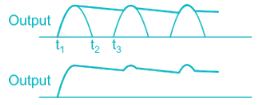Output DC voltage  Vavg = V= √2 × 230
= 325.3 V

The load current is given by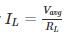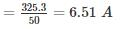This current discharges the capacitor to the load resistance during the interval (t2 to t3)

The time period of AC voltage.

= 20 ms (for 50 Hz)

Thus the charge supplied by the capacitor to the load resistance during this interval will be

∆Q = ILT = 6.51 × 20 × 10-3

= 0.1302 coulombs

Peak to peak ripple voltage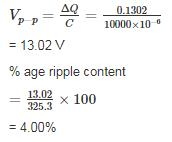QUESTION: 8

For the diode network shown below is input with voltage pulse Vi. If the knee voltage of the diode is Vk = 0.7 V then the output voltage pulse Vo is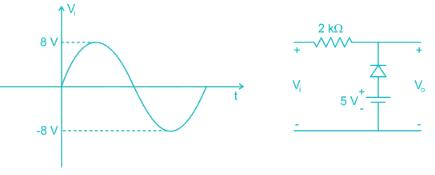Solution:

Given circuit can be written with ideal diode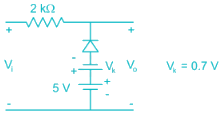Is input with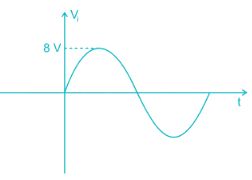During positive values of Vi < s – Vk i.e. Vi < 4.3 V the diode is in on-state, the diode can be taken as short circuit. The output voltage is simply be voltage across 5V voltage source.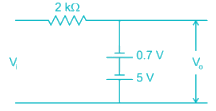⇒ Vo = s – 0.7 V

= 4.3 V

For Vi > 4.3 V the diode is in off-state the circuit become open circuit hence Vo = Vi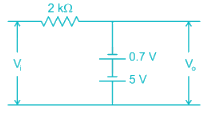The circuit is in on-state for entire negative cycle. Hence Vo = 4.3 V

The wave form of output voltage is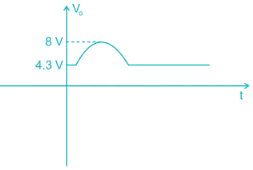*Answer can only contain numeric values
QUESTION: 9

In Fig. the Input Vis 100 Hz triangular wave having a peak to peak amplitude of 2 volts and an average value of 0 volts. Given that the diode is ideal, the average value of output Vo is ______

Solution:

Time period = 1/f = 1/100 = 10msec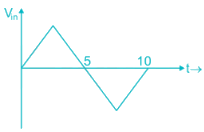1) During +Ve ½ cycle

Vin = + Ve diode is not forward bias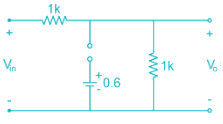2) During – Ve cycle

Vin = - Ve diode is Reverse Bias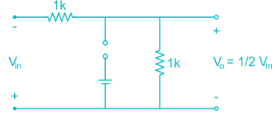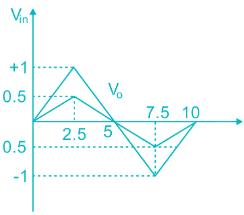Average value  = 0

*Answer can only contain numeric values
QUESTION: 10

In the circuit shown I is a dC current and Vi is a sinusoidal signal with peak amplitude 10 mV and a frequency 100 kHz. The value of I that will provide a phase shift of -45° at the output is ________. μA (Assume η = 1)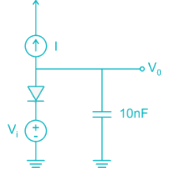Solution:

Opening the current source we get the AC small-signal circuit (η = 1)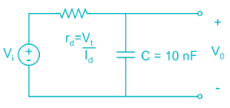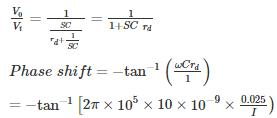I = 157 uA